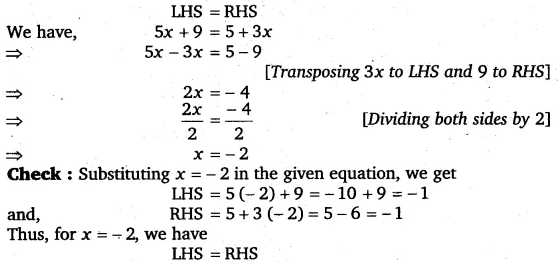# Solve the following equations and check your results: 5x + 9 = 5 + 3x

Solve the following equations and check your results:
5x + 9 = 5 + 3x